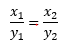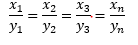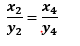# Direct and Inverse Proportion

## Introduction

We come across many such situations in our day-to-day life, where we need to see variation in one quantity bringing in variation in the other quantity.
For example:
(i) As the speed of a vehicle increases, the time taken to cover the same distance decreases
(ii) More apples cost more money
(iii) More interest earned for more money deposited.
(iv) More distance to travel, more petrol needed.

## Direct Proportion

Two quantities x and y are said to be in direct proportion
if they increase (decrease) together in such a manner that the ratio of their corresponding values remains constant.
That is if x/y=k= [k is a positive number] = Constant
Then x and y are said to vary directly. In such a case if y1, y2 are the values of y corresponding to the values x1, x2 of x respectively then## How to Solve Direct Proportion Problems

Two method to solve the problem
1) We know that in direct ProportionIn a problem, one ratio would be given. So using the above equation, we can easily find the unknown terms
Example
The cost of 5 kg of a particular quality of sugar is Rs 200. Tabulate the cost of 1,2, 4, 10 and 14   kg of sugar of the same type
Solution
Let x kg of sugar cost y rupees
 x 1 2 4 5 10 14 y ? ? ? 200 ? ?

As the number of kg increases, cost of the sugar also increases in the same ratio. It is case of direct proportion.
Now let’s use the above expressionNow we have
a)

• 1/y = 5/200
• y=Rs 40
b)

##• 2/y = 5/200
• y=Rs 80
Similarly, other can be found
Complete table would be

 x 1 2 4 5 10 14 y 40 80 160 200 400 560

2) We know that in direct Proportion
x/y= k
• x=ky
So we can find value of k from known values, and then use the formula to calculate the unknown values

## Inverse proportion

Two quantities x and y are said to be in inverse proportion

if an increase in x causes a proportional decrease in y (and vice-versa) in such a manner that the product of their corresponding values remains constant.
That is, if xy = k= Constant
Then x and y are said to vary inversely.
In this case if y1, y2 are the values of y corresponding to the values x1, x2 of x respectively then
x1 y1 = x2 y2

## How to Solve Inverse Proportion Problems

Two method to solve the problem
1) We know that in inverse Proportion
x1 y1 = x2 y2 = x2 y2 = x2 y2
In a problem, one pair would be given. So using the above equation, we can easily find the unknown terms
2) We know that in inverse Proportion
xy= k
• x=k/y
So we can find value of k from known values, and then use the formula to calculate the unknown values
Example:
If 20 workers can build a wall in 48 hours, how many workers will be required to do the same work in 30 hours?
Solution:
Let the number of workers employed to build the wall in 30 hours be y.
We have the following table.
 Number of Hours 48 30 Number of workers 20 y

Obviously more the number of workers, faster will they build the wall.
So, the number of hours and number of workers vary in inverse proportion.
So 48 × 20 = 30 × y
y=32 workers
So  to finish the work in 30 hours, 32 workers are required

## Solved Example

Example
Following are the car parking charges near an Airport up to
2 hours Rs 60
6 hours Rs 100
12 hours Rs 140
24 hours Rs 180
Check if the parking charges are in direct proportion to the parking time.
We know that two quantities are in direct proportion if whenever the values of one quantity increase, then the value of another quantity increase in such a way that ratio of the quantities remains same
Here The charges are not increasing in direct proportion to the parking time because
2/60 ≠ 6/100 ≠ 12/140 ≠ 24/180
Extra Zing
When two quantities x and y are in direct proportion (or vary directly) they are also written as xy.
When two quantities x and y are in inverse proportion (or vary inversely) they are also written as x∝ 1/y

Reference Books for class 8 Math

Given below are the links of some of the reference books for class 8 Math.

1. Mathematics Foundation Course for JEE/Olympiad : Class 8 This book can take students maths skills further. Only buy if child is interested in Olympiad/JEE foundation courses.
2. Mathematics for Class 8 by R S Aggarwal Detailed Mathematics book to clear basics and concepts. I would say it is a must have book for class 8 student.
3. Pearson Foundation Series (IIT -JEE / NEET) Physics, Chemistry, Maths & Biology for Class 8 (Main Books) | PCMB Combo : These set of books could help your child if he aims to get extra knowledge of science and maths. These would be helpful if child wants to prepare for competitive exams like JEE/NEET. Only buy if you can provide help to the child while studying.
4. Reasoning Olympiad Workbook - Class 8 :- Reasoning helps sharpen the mind of child. I would recommend students practicing reasoning even though they are not appearing for Olympiad.

You can use above books for extra knowledge and practicing different questions.

Note to our visitors :-

Thanks for visiting our website. From feedback of our visitors we came to know that sometimes you are not able to see the answers given under "Answers" tab below questions. This might happen sometimes as we use javascript there. So you can view answers where they are available by reloding the page and letting it reload properly by waiting few more seconds before clicking the button.
We really do hope that this resolve the issue. If you still hare facing problems then feel free to contact us using feedback button or contact us directly by sending is an email at [email protected]
We are aware that our users want answers to all the questions in the website. Since ours is more or less a one man army we are working towards providing answers to questions available at our website.